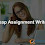Recently I have created demo at http://www.algorithmdemos.com/trees/binary-Search-Tree.html to explain how Binary Search Tree works. Initially to draw binary search tree, I have used equal distance between nodes, Then I have seen overlapping of nodes as shown in below diagram.
Every node position will be based on its parent node position. If parent node is at (300, 0)  then its child positions will be (250, 50) and (350, 50). Here X coordinate incremented or decremented by 50 and Y coordinate incremented by 50. Because of this approach I got overlapping problem as shown in above diagram. ( Node 6, 10 overlapping in the above diagram).

In Balanced Binary Tree, nodes will increase in exponential way along with height. So we can decide X -coordinate of  Tree Node using exponential decay. X coordinate factor will be decreased in exponential way
HeightNumber of NodesExponent (2 (height - 1) = number of nodes)X coordinate factor
110NA
221xfactor X (1/2)1
342xfactor X (1/2)2
483xfactor X (1/2)3

Observe the below diagram. Here I have taken X - factor as 300. The nodes were not overlapped because of exponential decay in distance.

1.This is quite a .good blog. Keep sharing. I love them Are you also searching for Nursing Writing Center? we are the best solution for you. We are best known for delivering nursing writing services to students without having to break the bank.

2.This comment has been removed by the author.

## BlogrollSrinivas Dasari find me on facebook follow me on twitter